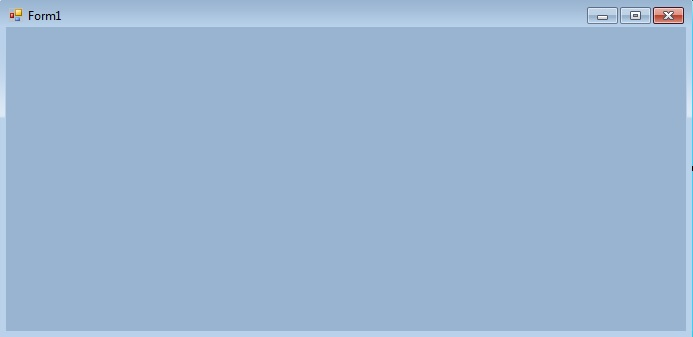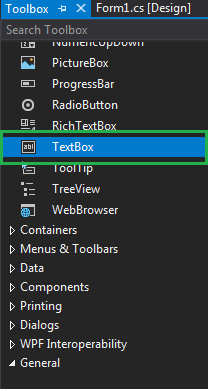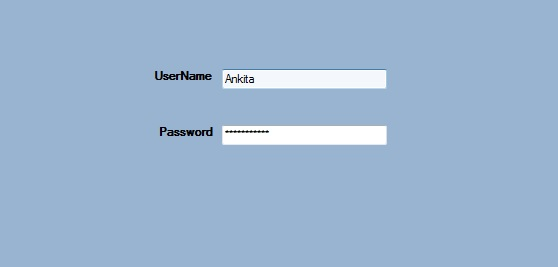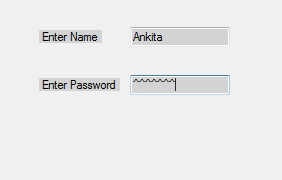# How to set the PasswordChar of the TextBox in C#?

• Last Updated : 29 Nov, 2019

In Windows forms, TextBox plays an important role. With the help of TextBox, the user can enter data in the application, it can be of a single line or of multiple lines. In TextBox, you are allowed to set the character used to mask characters of a password in a single-line TextBox control with the help of PasswordChar property of the TextBox. When the property is set, you are not allowed to use cut and copy actions in the control using the keyboard. In Windows form, you can set this property in two different ways:

1. Design-Time: It is the simplest way to set the PasswordChar property of the TextBox. As shown in the following steps:

• Step 1: Create a windows form. As shown in the below image:
Visual Studio -> File -> New -> Project -> WindowsFormApp• Step 2: Drag the TextBox control from the ToolBox and Drop it on the windows form. You can place TextBox anywhere on the windows form according to your need. As shown in the below image:• Step 3: After drag and drop you will go to the properties of the TextBox control to set the PasswordChar property of the TextBox. As shown in the below image:Output:2. Run-Time: It is a little bit trickier than the above method. In this method, you can set the PasswordChar property of the TextBox programmatically with the help of given syntax:

`public char PasswordChar { get; set; }`

Here, the value of this property is of System.Char type and the character used to mask characters entered in a single-line TextBox. The value of this property is set to 0 (character value) if you do not want the control to mask characters as they are typed. The default value of this property is Equals 0 (character value). Following steps are used to set the PasswordChar property of the TextBox:

• Step 1 : Create a textbox using the TextBox() constructor provided by the TextBox class.
```// Creating textbox
TextBox Mytextbox = new TextBox();
```
• Step 2 : After creating TextBox, set the PasswordChar property of the TextBox provided by the TextBox class.
```// Set PasswordChar property
```
• Step 3 : And last add this textbox control to from using Add() method.
```// Add this textbox to form
```

Example:

 `using` `System;``using` `System.Collections.Generic;``using` `System.ComponentModel;``using` `System.Data;``using` `System.Drawing;``using` `System.Linq;``using` `System.Text;``using` `System.Threading.Tasks;``using` `System.Windows.Forms;`` ` `namespace` `my {`` ` `public` `partial` `class` `Form1 : Form {`` ` `    ``public` `Form1()``    ``{``        ``InitializeComponent();``    ``}`` ` `    ``private` `void` `Form1_Load(``object` `sender, EventArgs e)``    ``{``        ``// Creating and setting the properties of Lable1``        ``Label Mylablel1 = ``new` `Label();``        ``Mylablel1.Location = ``new` `Point(96, 54);``        ``Mylablel1.Text = ``"Enter Name"``;``        ``Mylablel1.AutoSize = ``true``;``        ``Mylablel1.BackColor = Color.LightGray;`` ` `        ``// Add this label to form``        ``this``.Controls.Add(Mylablel1);`` ` `        ``// Creating and setting the properties of TextBox1``        ``TextBox Mytextbox1 = ``new` `TextBox();``        ``Mytextbox1.Location = ``new` `Point(187, 51);``        ``Mytextbox1.BackColor = Color.LightGray;``        ``Mytextbox1.AutoSize = ``true``;``        ``Mytextbox1.Name = ``"text_box1"``;`` ` `        ``// Add this textbox to form``        ``this``.Controls.Add(Mytextbox1);`` ` `        ``// Creating and setting the properties of Lable1``        ``Label Mylablel2 = ``new` `Label();``        ``Mylablel2.Location = ``new` `Point(96, 102);``        ``Mylablel2.Text = ``"Enter Password"``;``        ``Mylablel2.AutoSize = ``true``;``        ``Mylablel2.BackColor = Color.LightGray;`` ` `        ``// Add this label to form``        ``this``.Controls.Add(Mylablel2);`` ` `        ``// Creating and setting the properties of TextBox2``        ``TextBox Mytextbox2 = ``new` `TextBox();``        ``Mytextbox2.Location = ``new` `Point(187, 99);``        ``Mytextbox2.BackColor = Color.LightGray;``        ``Mytextbox2.AutoSize = ``true``;``        ``Mytextbox2.PasswordChar = ``'^'``;``        ``Mytextbox2.Name = ``"text_box2"``;`` ` `        ``// Add this textbox to form``        ``this``.Controls.Add(Mytextbox2);``    ``}``}``}`

Output:• My Personal Notes arrow_drop_up